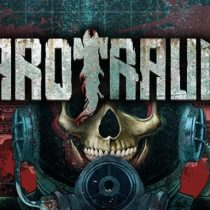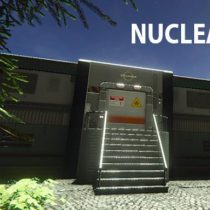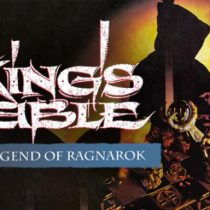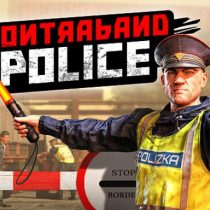#### Simulation GAMES• Action
• Shooter
• Sci-fi
• Open World
• Simulation• Survival
• Simulation
• Sci-fi
• Horror
• Strategy
• Management
• Action• Nudity
• Mature
• RPG
• Simulation
• Visual Novel• Simulation
• RPG
• Casual
• Visual Novel
• Mature
• Anime• Management
• Sci-fi
• Strategy
• Simulation
• Building• Simulation
• Strategy
• Action
• Sci-fi• Casual
• Strategy
• Management
• Simulation• Strategy
• Management
• Casual
• Simulation• Casual
• Racing
• Simulation• Strategy
• Simulation
• Building
• Management• Simulation
• Strategy
• Action
• Anime• RPG
• Simulation
• Visual Novel
• Strategy• Simulation
• Nudity
• Casual
• Mature
• Anime
• RPG• Action
• Open World
• Survival
• Management
• Simulation• Management
• Simulation
• Building
• Strategy• Simulation
• Casual
• Strategy
• Management
• Anime
• RPG• Action
• Casual
• Simulation• Horror
• RPG
• Simulation• Management
• Simulation
• RPG
• Open World